Courses

# Comprehension Based Questions Of Aldehydes, Ketones And Carboxylic Acids, Past Year Questions JEE Advance, Class 12, Chemistry

## 19 Questions MCQ Test Class 12 Chemistry 35 Years JEE Mains &Advance Past yr Paper | Comprehension Based Questions Of Aldehydes, Ketones And Carboxylic Acids, Past Year Questions JEE Advance, Class 12, Chemistry

Description
This mock test of Comprehension Based Questions Of Aldehydes, Ketones And Carboxylic Acids, Past Year Questions JEE Advance, Class 12, Chemistry for JEE helps you for every JEE entrance exam. This contains 19 Multiple Choice Questions for JEE Comprehension Based Questions Of Aldehydes, Ketones And Carboxylic Acids, Past Year Questions JEE Advance, Class 12, Chemistry (mcq) to study with solutions a complete question bank. The solved questions answers in this Comprehension Based Questions Of Aldehydes, Ketones And Carboxylic Acids, Past Year Questions JEE Advance, Class 12, Chemistry quiz give you a good mix of easy questions and tough questions. JEE students definitely take this Comprehension Based Questions Of Aldehydes, Ketones And Carboxylic Acids, Past Year Questions JEE Advance, Class 12, Chemistry exercise for a better result in the exam. You can find other Comprehension Based Questions Of Aldehydes, Ketones And Carboxylic Acids, Past Year Questions JEE Advance, Class 12, Chemistry extra questions, long questions & short questions for JEE on EduRev as well by searching above.
QUESTION: 1

### PASSAGE -1 In the following reaction sequence, product I, J and L are formed. K represents a reagent.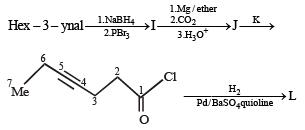Q.The structure of the product I is –

Solution: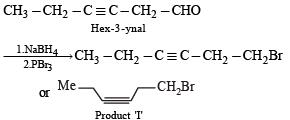Sodium borohydride reduces –CHO Selectively into –CH2O

QUESTION: 2

### In the following reaction sequence, product I, J and L are formed. K represents a reagent.Q. The structures of compound J and K, respectively, are

Solution: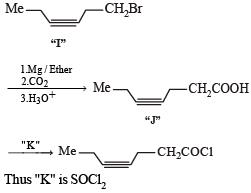QUESTION: 3

### In the following reaction sequence, product I, J and L are formed. K represents a reagent.Q. The structure of product L is

Solution: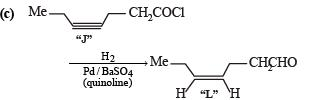It is Rosemmund reaction. Simultaneously the reagent H2-Pd also reduces carbon-carbon triple bond to double bond (syn -addition) giving cis product.

QUESTION: 4

PASSAGE-2

A carbonyl compound P,  which gives positive iodoform test, undergoes reaction with MeMgBr followed by dehydration to give an olefin Q. Ozonolysis of Q leads to a dicarbonyl compound R, which undergoes intramolecular aldol reaction to give predominantly S.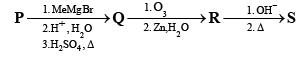Q. The structure of the carbonyl compound P is

Solution:

Iodoform test of compound P points out that P has – COCH3 group which shows that it may be either option (a) or (b) of Q. 16. Further since the dicarbonyl compound R has at least one a-H atom w.r.t to one of the carbonyl groups which is possible when R is produced from (b) of Q. 18; (a) option of Q. 16 will give dicarbonyl compound having two –CHO, none of which has a-H atom.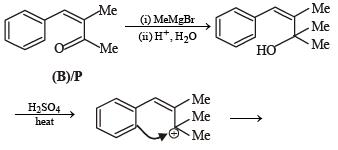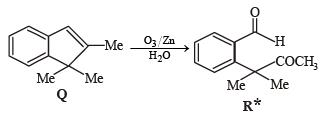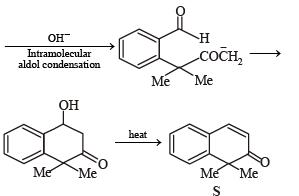* Structure of R would be R' when P is (A)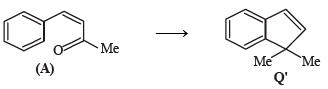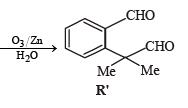QUESTION: 5

PASSAGE-2

A carbonyl compound P,  which gives positive iodoform test, undergoes reaction with MeMgBr followed by dehydration to give an olefin Q. Ozonolysis of Q leads to a dicarbonyl compound R, which undergoes intramolecular aldol reaction to give predominantly S.Q. The structures of the products Q and R, respectively, are

Solution:

Iodoform test of compound P points out that P has – COCH3 group which shows that it may be either option (a) or (b) of Q. 16. Further since the dicarbonyl compound R has at least one a-H atom w.r.t to one of the carbonyl groups which is possible when R is produced from (b) of Q. 18; (a) option of Q. 16 will give dicarbonyl compound having two –CHO, none of which has a-H atom.* Structure of R would be R' when P is (A)QUESTION: 6

PASSAGE-2

A carbonyl compound P,  which gives positive iodoform test, undergoes reaction with MeMgBr followed by dehydration to give an olefin Q. Ozonolysis of Q leads to a dicarbonyl compound R, which undergoes intramolecular aldol reaction to give predominantly S.Q. The structure of the product S is

Solution:

Iodoform test of compound P points out that P has – COCH3 group which shows that it may be either option (a) or (b) of Q. 16. Further since the dicarbonyl compound R has at least one a-H atom w.r.t to one of the carbonyl groups which is possible when R is produced from (b) of Q. 18; (a) option of Q. 16 will give dicarbonyl compound having two –CHO, none of which has a-H atom.* Structure of R would be R' when P is (A)QUESTION: 7

PASSAGE-3

Two aliphatic aldehydes P and Q react in the presence of aqueous K2CO3 to give compound R, which upon treatment with HCN provides compound S. On acidification and heating, S gives the product shown below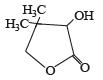Q. The compounds P and Q respectively are :

Solution:

Let us summarize the given facts of the problem.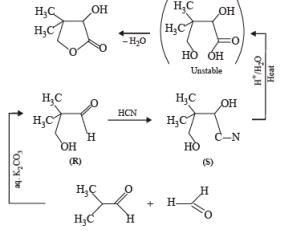(P & Q)

QUESTION: 8

PASSAGE-3

Two aliphatic aldehydes P and Q react in the presence of aqueous K2CO3 to give compound R, which upon treatment with HCN provides compound S. On acidification and heating, S gives the product shown below.Q. The compound R is :

Solution:

Let us summarize the given facts of the problem.(P & Q)

QUESTION: 9

PASSAGE-3

Two aliphatic aldehydes P and Q react in the presence of aqueous K2CO3 to give compound R, which upon treatment with HCN provides compound S. On acidification and heating, S gives the product shown below.

Q. The compound S is :

Solution:

Let us summarize the given facts of the problem.(P & Q)

QUESTION: 10

PASSAGE-4

In the following reaction sequence, the compound J is an intermediate.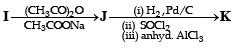J (C9H8O2) gives effervescence on treatment with NaHCO3 and a positive Baeyer’s test.

Q. The compound I is

Solution:

Reactions of compound J (C9H8O2) indicates that  it has C = C linkage and – COOH group. Thus, J can be written as C6H5CH = CH COOH. Since, J is unsaturated carboxylic acid and it is formed by the reactions of compound I with (CH3CO)2O and CH3COONa, compound I should be an aldehyde (recall Perkin reaction). Thus the whole series of reactions can be written as below.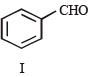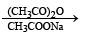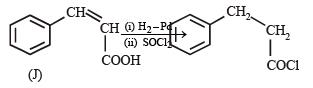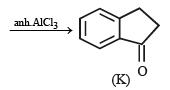QUESTION: 11

PASSAGE-4

In the following reaction sequence, the compound J is an intermediate.J (C9H8O2) gives effervescence on treatment with NaHCO3 and a positive Baeyer’s test.

Q. The compound K is

Solution:

Reactions of compound J (C9H8O2) indicates that  it has C = C linkage and – COOH group. Thus, J can be written as C6H5CH = CH COOH. Since, J is unsaturated carboxylic acid and it is formed by the reactions of compound I with (CH3CO)2O and CH3COONa, compound I should be an aldehyde (recall Perkin reaction). Thus the whole series of reactions can be written as below.QUESTION: 12

PASSAGE-5

P and Q are isomers of dicarboxylic acid C4H4O4. Both decolorize Br2/H2O. On heating, P forms the cyclic anhydride. Upon treatment with dilute alkaline KMnO4, P as well as Q could produce one or more than one from S, T and U.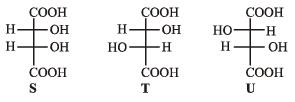Solution: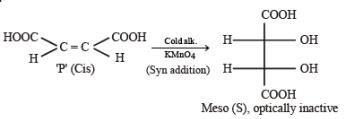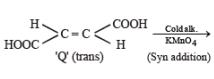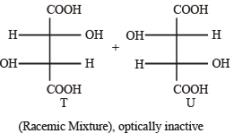QUESTION: 13

PASSAGE-5

P and Q are isomers of dicarboxylic acid C4H4O4. Both decolorize Br2/H2O. On heating, P forms the cyclic anhydride. Upon treatment with dilute alkaline KMnO4, P as well as Q could produce one or more than one from S, T and U.Q. In the following reaction sequences V and W are respectively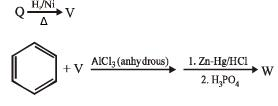Solution: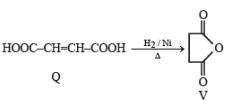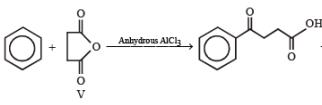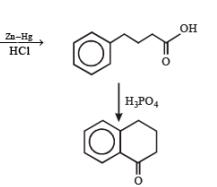QUESTION: 14

PASSAGE-6

In the following reactions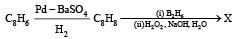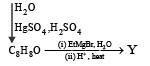Q. Compound X is

Solution: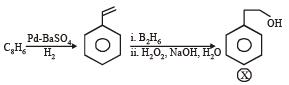QUESTION: 15

PASSAGE-6

In the following reactionsQ. The major compound Y is

Solution: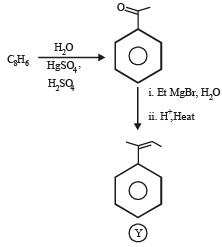QUESTION: 16

Each of  this question contains STATEMENT-1 (Assertion/ Statement ) and STATEMENT-2 (Reason/Explanation) and has 4 choices (a), (b), (c) and (d) out of which ONLY ONE is correct.

Q.

Statement-1 : Acetate ion is more basic than the methoxide ion.

Statement-2 : The acetate ion is resonance stabilized

Solution:

TIPS/Formulae :

Acetate ion is reasonance stabilized while methoxide ion is not.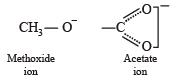Hence, acetate ion is less basic than methoxide ion.

QUESTION: 17

Statement-1 : Acetic acid does not undergo haloform reaction.

Statement-2 : Acetic acid has no alpha hydrogens.

Solution:

Haloform reaction is undergone only by ketones, CH3COOH has 3 α-hydrogens.

QUESTION: 18

Statement-1 : Dimethyl sulphide is commonly used for the reduction of an ozonide of an alkene to get the carbonyl compounds.

Statement-2 : It reduces the ozonide giving water soluble dimethyl sulphoxide and excess of it evaporates.

Solution:

TIPS/Formulae : Ozonide can be reduced by (CH3)2S to give carbonyl compounds and dimethyl sulphoxide.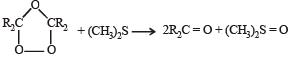QUESTION: 19

Statement-1 : p-Hydroxybenzoic acid has a lower boiling point than o-hydroxybenzoic acid.

Statement-2 : o-Hydroxybenzoic acid has intramolecular hydrogen bonding.

Solution:

p-Hydroxybenzoic acid has higher boiling point than o-hydroxybenzoic acid due to intermolecular hydrogen bonding. Thus, statement-1 is false. o-Hydroxybezoic acid shows intramolecular H-bonding thus, statement2 is true.